# Texas Go Math Grade 4 Lesson 3.6 Answer Key Rename Fractions and Mixed Numbers

Refer to our Texas Go Math Grade 4 Answer Key Pdf to score good marks in the exams. Test yourself by practicing the problems from Texas Go Math Grade 4 Lesson 3.6 Answer Key Rename Fractions and Mixed Numbers.

## Texas Go Math Grade 4 Lesson 3.6 Answer Key Rename Fractions and Mixed Numbers

Essential Question

How can you rename mixed numbers as fractions greater than I and rename fractions greater than I as mixed numbers?

Unlock the Problem

Mr. Fox has 2$$\frac{3}{6}$$ loaves of corn bread. Each loaf was cut into $$\frac{1}{6}$$-size pieces. If he has 14 people over for dinner, is there enough bread for each person to have 1 piece?

• What is the size of 1 piece of bread relative to the whole?

A mixed number is a number represented by a whole number and a fraction. You can write a mixed number as a fraction. To find how many $$\frac{1}{6}$$-size pieces are in 2$$\frac{3}{6}$$ write 2$$\frac{3}{6}$$ as a fraction.

• How much bread does Mr. Fox need for 14 people?

Example: Write a mixed number as a fraction.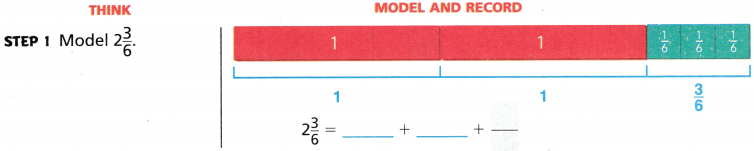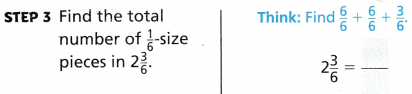There are __________ sixth-size pieces in 2$$\frac{3}{6}$$.
So, there is enough bread for 14 people to each have 1 piece.

Example Write a fraction greater than 1 as a mixed number.

To weave a bracelet, Charlene needs 7 pieces of brown thread. Each piece of thread must be $$\frac{1}{3}$$ yard long. How much thread should she buy to weave the bracelet?

Write $$\frac{7}{3}$$ as a mixed number.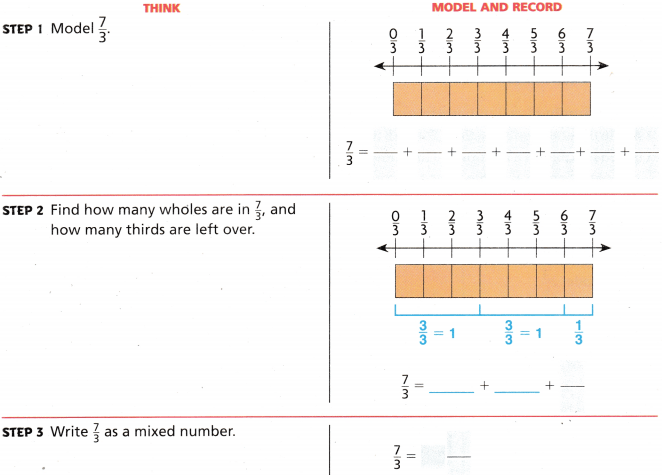Share and Show

Write the unknown numbers. Write mixed numbers above the number line and fractions greater than one below the number line.

Question 1.Write the mixed number as a fraction or the fraction as a mixed number.

Question 2.
1$$\frac{1}{8}$$ ______________

Rename Fractions and Mixed Numbers 4th Grade Question 3.
$$\frac{13}{10}$$ ______________

Question 4.
1$$\frac{2}{3}$$ ______________

Math Talk

Mathematical Processes
Describe how you can compare 1$$\frac{3}{5}$$ and $$\frac{7}{5}$$.

H.O.T. Algebra Find the unknown numbers.

Question 5.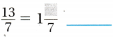Question 6.Question 7.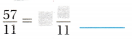Problem Solving

Use the recipe to solve 8-10.Question 8.
Cal is making energy squares. How many  cups of peanut butter are used in the recipe?

Question 9.
What is the amount of bran cereal, written as a fraction greater than 1, that is used in the recipe?

Question 10.
H.O.T. Apply Suppose Cal wants to make 2 times as many energy squares as the recipe makes. How many cups of bran cereal should he use? Write your answer as a mixed number and as a fraction greater than 1 in simplest form.

Question 11.
Cal added 2$$\frac{3}{8}$$ cups of raisins. Write this mixed number as a fraction greater than 1 in simplest form.

Question 12.
H.O.T. Multi-Step Jenn is preparing brown rice. She needs 1$$\frac{1}{2}$$ cups of brown rice and 2 cups of water. Jenn has only a $$\frac{1}{8}$$-cup measuring cup. How many $$\frac{1}{8}$$ cups each of rice and water will Jenn use to prepare the rice?

Lesson 3.6 Rename Fractions and Mixed Numbers Question 13.
Multi-Step Pen has $$\frac{1}{2}$$-cup and $$\frac{1}{8}$$-cup measuring cups. What are two ways he could measure out 1$$\frac{3}{4}$$ cups of flour?

Question 14.
Use Tools The weekly grocery list for a zoo includes 2$$\frac{3}{5}$$ pounds of crickets. What is 2$$\frac{3}{5}$$ written as a fraction?(A) $$\frac{10}{5}$$
(B) $$\frac{3}{5}$$
(C) $$\frac{13}{5}$$
(D) $$\frac{1}{5}$$

Question 15.
Derrick needs 4 strips of wood to make a frame. Each strip must be $$\frac{1}{3}$$ yard long. How much wood should Derrick buy to make the frame?
(A) 1 yard
(B) 1$$\frac{4}{3}$$ yards
(C) $$\frac{1}{3}$$ yard
(D) 1$$\frac{1}{3}$$ yards

Question 16.
A box of cereal contains 4$$\frac{3}{4}$$ cups of cereal. Each serving is $$\frac{1}{4}$$ cup. How many servings of cereal are in the box?
(A) 16
(B) 19
(C) 4
(D) 3

TEXAS Test Prep

Question 17.
Which fraction greater than 1 can you write for 4$$\frac{5}{9}$$?
(A) $$\frac{9}{9}$$
(B) $$\frac{18}{9}$$
(C) $$\frac{41}{9}$$
(D) $$\frac{45}{9}$$

### Texas Go Math Grade 4 Lesson 3.6 Homework and Practice Answer Key

Write the unknown numbers. Write mixed numbers above the number line and fractions greater than one below the number line.

Question 1.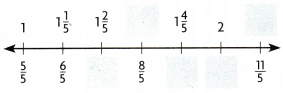Write the mixed number as a fraction.

Question 2.
2$$\frac{1}{4}$$ ____________

Question 3.
3$$\frac{2}{3}$$ ____________

Question 4.
4$$\frac{1}{6}$$ ____________

Write the fraction as a mixed number.

Question 5.
$$\frac{7}{6}$$ ____________

Rename Fractions and Mixed Numbers Lesson 3.6 Question 6.
$$\frac{14}{2}$$ ____________

Question 7.
$$\frac{8}{3}$$ ____________

Problem Solving

Use the recipe to solve 8-9.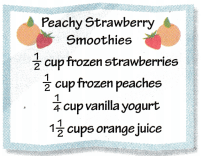Question 8.
Celia is making smoothies. How many $$\frac{1}{4}$$ cups of peaches are used in the recipe?

Question 9.
What is the amount of orange juice, written as a fraction greater than 1, that is used in the recipe?

Lesson Check

Question 10.
Calvin is making birdhouses to sell at the tail. So far he has finished 5$$\frac{1}{2}$$ bird houses. Which fraction greater than one represents this mixed number?
(A) $$\frac{10}{3}$$
(B) $$\frac{6}{2}$$
(C) $$\frac{5}{3}$$
(D) $$\frac{11}{3}$$

Question 11.
Which mixed number can you write for $$\frac{24}{7}$$?
(A) 24$$\frac{1}{7}$$
(B) 4$$\frac{3}{7}$$
(C) 3$$\frac{3}{7}$$
(D) 3$$\frac{1}{7}$$

Question 12.
Paolo uses 3$$\frac{1}{4}$$ cups of oatmeal to make breakfast bars. What fraction greater than one represents this mixed number?
(A) $$\frac{13}{4}$$
(B) $$\frac{12}{5}$$
(C) $$\frac{4}{4}$$
(D) $$\frac{31}{4}$$

Question 13.
Which mixed number can you write for $$\frac{13}{5}$$
(A) 1$$\frac{3}{5}$$
(B) 13$$\frac{1}{5}$$
(C) 3$$\frac{2}{5}$$
(D) 2$$\frac{3}{5}$$

Question 14.
Multi-Step Juanita is making bread. She needs 3$$\frac{1}{2}$$ cups of flour. Juanita only has a $$\frac{1}{4}$$ cup measuring cup. How many $$\frac{1}{4}$$ cups of flour will Juanita use to prepare the bread?
(A) 14
(B) 7
(C) 9
(D) 12
Multi-Step Each day for 7 days, Mr. Cole used $$\frac{1}{2}$$ can of paint. Which mixed number represents how much paint Mr. Cole used after 7 days?
(A) 7$$\frac{1}{2}$$
(B) 2$$\frac{1}{2}$$
(C) 3$$\frac{1}{2}$$
(D) 8$$\frac{1}{2}$$